0%

Note - These are my notes on DeepLearning Specialization Part:
Regularization || Deeplearning (Course - 2 Week - 1) || Improving Deep Neural Networks(Week 1)

### Introduction:

And usually, you set this using your development set, or using [INAUDIBLE] cross validation. When you a variety of values and see what does the best, in terms of trading off between doing well in your training set versus also setting that two normal of your parameters to be small. Which helps prevent over fitting. So lambda is another hyper parameter that you might have to tune. And by the way, for the programming exercises, lambda is a reserved keyword in the Python programming language. So in the programming exercise, we’ll have lambd,
]without the a, so as not to clash with the reserved keyword in Python. So we use lambd to represent the lambda regularization parameter.

$$\text{minimize(Loss(Data|Model))}$$
we’ll now minimize loss+complexity, which is called structural risk minimization:

$$\text{minimize(Loss(Data|Model) + complexity(Model))}$$
Our training optimization algorithm is now a function of two terms: the loss term, which measures how well the model fits the data, and the regularization term, which measures model complexity.

Model complexity as a function of the weights of all the features in the model.
Model complexity as a function of the total number of features with nonzero weights. (A later module covers this approach.)
If model complexity is a function of weights, a feature weight with a high absolute value is more complex than a feature weight with a low absolute value.

We can quantify complexity using the L2 regularization formula, which defines the regularization term as the sum of the squares of all the feature weights:

$$L_2 \text{ regularization term} = ||\boldsymbol w||_2^2 = {w_1^2 + w_2^2 + … + w_n^2}$$

In this formula, weights close to zero have little effect on model complexity, while outlier weights can have a huge impact.

For example, a linear model with the following weights:

$${w_1 = 0.2, w_2 = 0.5, w_3 = 5, w_4 = 1, w_5 = 0.25, w_6 = 0.75}$$

Has an L2 regularization term of 26.915:

$$w_1^2 + w_2^2 + \boldsymbol{w_3^2} + w_4^2 + w_5^2 + w_6^2$$ $$= 0.2^2 + 0.5^2 + \boldsymbol{5^2} + 1^2 + 0.25^2 + 0.75^2$$ $$= 0.04 + 0.25 + \boldsymbol{25} + 1 + 0.0625 + 0.5625$$
$$= 26.915$$
But (w_3) (bolded above), with a squared value of 25, contributes nearly all the complexity. The sum of the squares of all five other weights adds just 1.915 to the L2 regularization term.

### Lambda Introduction

Model developers tune the overall impact of the regularization term by multiplying its value by a scalar known as lambda (also called the regularization rate). That is, model developers aim to do the following:

$$\text{minimize(Loss(Data|Model)} + \lambda \text{ complexity(Model))}$$
Performing L2 regularization has the following effect on a model

Encourages weight values toward 0 (but not exactly 0)
Encourages the mean of the weights toward 0, with a normal (bell-shaped or Gaussian) distribution.
Increasing the lambda value strengthens the regularization effect. For example, the histogram of weights for a high value of lambda
Some of your training examples of the losses of the individual predictions in the different examples, where you recall that w and b in the logistic regression, are the parameters. So w is an x-dimensional parameter vector, and b is a real number. And so to add regularization to the logistic regression, what you do is add to it this thing, lambda, which is called the regularization parameter. I’ll say more about that in a second. But lambda/2m times the norm of w squared. So here, the norm of w squared is just equal to sum from j equals 1 to nx of wj squared, or this can also be written w transpose w, it’s just a square Euclidean norm of the prime to vector w. And this is called L2 regularization.

When choosing a lambda value, the goal is to strike the right balance between simplicity and training-data fit:

If your lambda value is too high, your model will be simple, but you run the risk of underfitting your data. Your model won’t learn enough about the training data to make useful predictions.

If your lambda value is too low, your model will be more complex, and you run the risk of overfitting your data. Your model will learn too much about the particularities of the training data, and won’t be able to generalize to new data.

Note: Setting lambda to zero removes regularization completely. In this case, training focuses exclusively on minimizing loss, which poses the highest possible overfitting risk.
The ideal value of lambda produces a model that generalizes well to new, previously unseen data. Unfortunately, that ideal value of lambda is data-dependent, so you’ll need to do some tuning.

L2 Regularization and lambda (Regularization Parameter): relation
There’s a close connection between learning rate and lambda. Strong L2 regularization values tend to drive feature weights closer to 0. Lower learning rates (with early stopping) often produce the same effect because the steps away from 0 aren’t as large. Consequently, tweaking learning rate and lambda simultaneously may have confounding effects.

Early stopping means ending training before the model fully reaches convergence. In practice, we often end up with some amount of implicit early stopping when training in an online (continuous) fashion. That is, some new trends just haven’t had enough data yet to converge.

As noted, the effects from changes to regularization parameters can be confounded with the effects from changes in learning rate or number of iterations. One useful practice (when training across a fixed batch of data) is to give yourself a high enough number of iterations that early stopping doesn’t play into things.

### Implementing L2 Regularisation in a Neural Network

So this is how you implement L2 regularization for logistic regression. How about a neural network? In a neural network, you have a cost function(J) that's a function of all of your parameters, w, b through w[L], b[L], where capital L is the number of layers in your neural network. And so the cost function is this, sum of the losses, summed over your m training examples.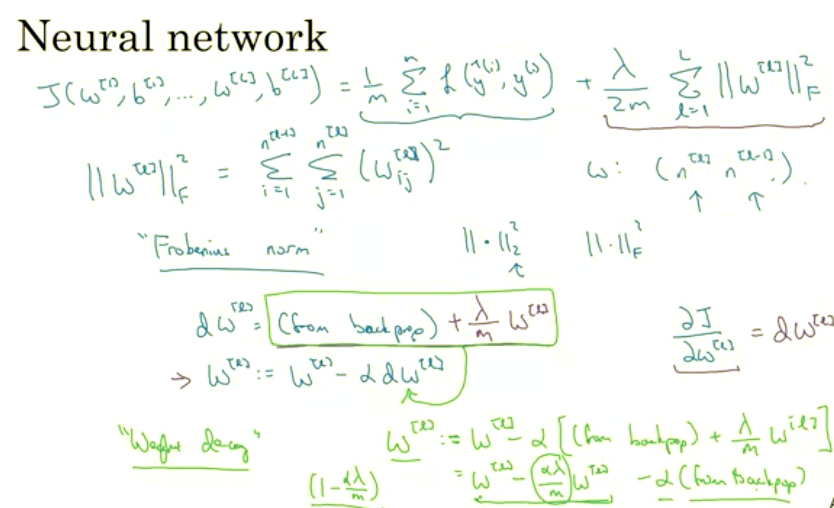#### L2 Norm ~ Weight Deacy

And it's for this reason that L2 regularization is sometimes also called weight decay. So if I take this definition of dw[l] and just plug it in here, then you see that the update is w[l] = w[l] times the learning rate alpha times the thing from backprop, +lambda of m times w[l]. Throw the minus sign there. And so this is equal to $$(w[l]- alpha * lambda / m) * (w[l]- alpha)$$ times the thing you got from backpop. And so this term shows that whatever the matrix w[l] is, you're going to make it a little bit smaller, right? This is actually as if you're taking the matrix w and you're multiplying it by $$1-alpha*lambda/m$$ . You're really taking the matrix w and subtracting $$alpha*lambda/m$$ with L2 Regularization penalty . Like you're multiplying matrix w by this number, which is going to be a little bit less than 1. So this is why L2 norm regularization is also called weight decay. Because it's just like the ordinally gradient descent, where you update w by subtracting alpha times the original gradient you got from backprop. But now you're also multiplying w by this thing, which is a little bit less than 1. So the alternative name for L2 regularization is weight decay. I'm not really going to use that name, but the intuition for it's called weight decay is that this first term here, is equal to this. So you're just multiplying the weight metrics by a number slightly less than 1. So that's how you implement L2 regularization in neural network. Now, one question that he has asked me is, why does regularization prevent over-fitting? Let's look at the next Section, and gain some intuition for how regularization prevents over-fitting.

### How do regularization Prevent Overfitting?

Why does regularization help with overfitting? Why does it help with reducing variance problems? Let's go through a couple examples to gain some intuition about how it works. So, recall that high bias, high variance. And I just write pictures from our earlier section that looks something like this.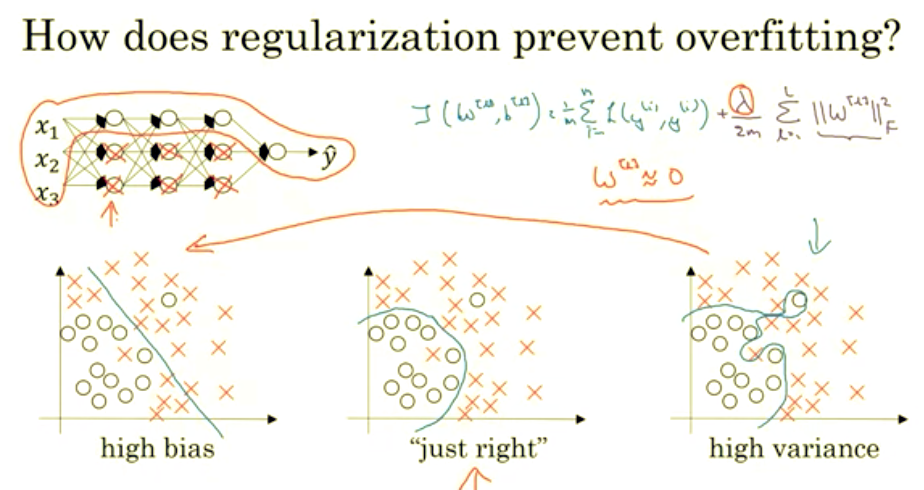Now, let's see a fitting large and deep neural network. I know I haven't drawn this one too large or too deep, unless you think some neural network and this currently overfitting. So you have some cost function like J of W, B equals sum of the losses.

$$\text{minimize(Loss(Data|Model)} + \lambda \text{ complexity(Model))}$$

So what we did for regularization was add this extra term that penalizes the weight matrices from being too large. So that was the Frobenius norm. So why is it that shrinking the L two norm or the Frobenius norm or the parameters might cause less overfitting? One piece of intuition is that if you crank regularisation lambda to be really, really big, they’ll be really incentivized to set the weight matrices W to be reasonably close to zero. So one piece of intuition is maybe it set the weight to be so close to zero for a lot of hidden units that’s basically zeroing out a lot of the impact of these hidden units. And if that’s the case, then this much simplified neural network becomes a much smaller neural network. In fact, it is almost like a logistic regression unit, but stacked most probably as deep. And so that will take you from this overfitting case much closer to the left to other high bias case. But hopefully there’ll be an intermediate value of lambda that results in a result closer to this just right case in the middle.
But the intuition is that by cranking up lambda to be really big they’ll set W close to zero, which in practice this isn’t actually what happens. We can think of it as zeroing out or at least reducing the impact of a lot of the hidden units so you end up with what might feel like a simpler network.
They get closer and closer as if you’re just using logistic regression.
The intuition of completely zeroing out of a bunch of hidden units isn’t quite right.
It turns out that what actually happens is they’ll still use all the hidden units, but each of them would just have a much smaller effect. But you do end up with a simpler network and as if you have a smaller network that is therefore less prone to overfitting.
So a lot of this intuition helps better when you implement regularization in the program exercise, you actually see some of these variance reduction results yourself.

### Another Intution with TanH as activation Function instead of Sigmoid

Here's another attempt at additional intuition for why regularization helps prevent overfitting. And for this, I'm going to assume that we're using the tanh activation function which looks like this.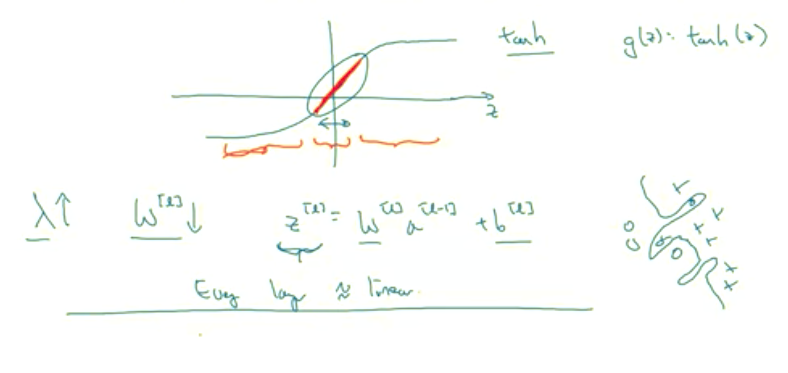This is a g of z equals tanh of z. So if that's the case, notice that so long as Z is quite small, so if Z takes on only a smallish range of parameters, maybe around here, then you're just using the linear regime of the tanh function. Is only if Z is allowed to wander up to larger values or smaller values like so, that the activation function starts to become less linear.

### Takeaway:

So the intuition you might take away from this is that if lambda, the regularization parameter, is large, then you have that your parameters will be relatively small, because they are penalized being large into a cos function. And so if the blades W are small then because Z is equal to W and then technically is plus b, but if W tends to be very small, then Z will also be relatively small. And in particular, if Z ends up taking relatively small values, just in this whole range, then G of Z will be roughly linear. So it's as if every layer will be roughly linear. As if it is just linear regression. And we saw in course one that if every layer is linear then your whole network is just a linear network. And so even a very deep network, with a deep network with a linear activation function is at the end they are only able to compute a linear function. So it's not able to fit those very very complicated decision. Very non-linear decision boundaries that allow it to really overfit right to data sets like we saw on the overfitting high variance case on the previous slide.

#### So just to summarize

If the regularization becomes very large, the parameters W very small, so Z will be relatively small, kind of ignoring the effects of b for now, so Z will be relatively small or, really,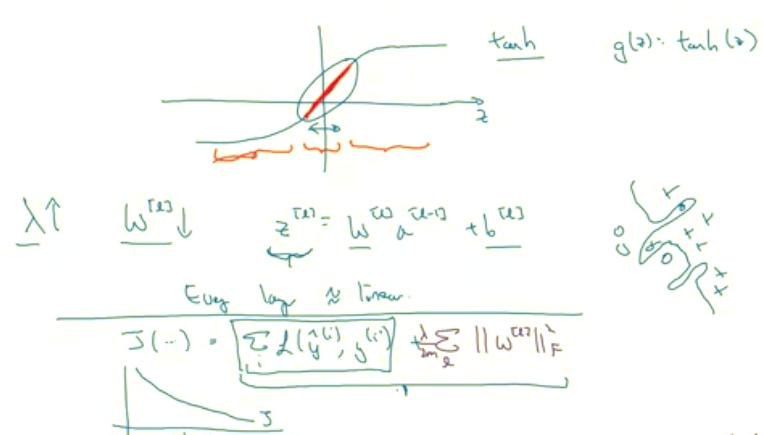I should say it takes on a small range of values. And so the activation function if is tanh, say, will be relatively linear. And so your whole neural network will be computing something not too far from a big linear function which is therefore pretty simple function rather than a very complex highly non-linear function. And so is also much less able to overfit. And again, when you enter in regularization for yourself in the program exercise, you'll be able to see some of these effects yourself.

#### Tip

Before wrapping up our def discussion on regularization, I just want to give you one implementational tip. Which is that, when implanting regularization, we took our definition of the cost function J and we actually modified it by adding this extra term that penalizes the weight being too large. And so if you implement gradient descent, one of the steps to debug gradient descent is to plot the cost function J as a function of the number of elevations of gradient descent and you want to see that the cost function J decreases monotonically after every elevation of gradient descent. And if you're implementing regularization then please remember that J now has this new definition. If you plot the old definition of J, just this first term, then you might not see a decrease monotonically. So to debug gradient descent make sure that you're plotting this new definition of J that includes this second term as well. Otherwise you might not see J decrease monotonically on every single elevation. So that's it for L two regularization which is actually a regularization technique that I use the most in training deep learning modules. In deep learning there is another sometimes used regularization technique called dropout regularization. Let's take a look at that in the next section.

### Dropout Regularization

In addition to L2 regularization, another very powerful regularization techniques is called "dropout." Let's see how that works. Let's say you train a neural network like the one on the left and there's over-fitting. Here's what you do with dropout. Let me make a copy of the neural network.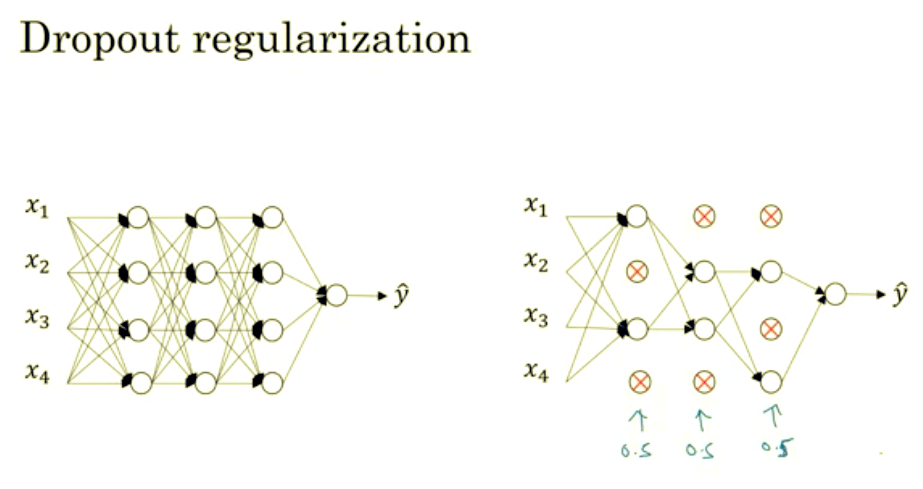With dropout, what we're going to do is go through each of the layers of the network and set some probability of eliminating a node in neural network. Let's say that for each of these layers, we're going to- for each node, toss a coin and have a 0.5 chance of keeping each node and 0.5 chance of removing each node. So, after the coin tosses, maybe we'll decide to eliminate those nodes, then what you do is actually remove all the outgoing things from that no as well. So you end up with a much smaller, really much diminished network. And then you do back propagation training. There's one example on this much diminished network. And then on different examples, you would toss a set of coins again and keep a different set of nodes and then dropout or eliminate different than nodes. And so for each training example, you would train it using one of these neural based networks. So, maybe it seems like a slightly crazy technique. They just go around coding those are random, but this actually works. But you can imagine that because you're training a much smaller network on each example or maybe just give a sense for why you end up able to regularize the network, because these much smaller networks are being trained.

### Implementing Dropout

Let's look at how you implement dropout. There are a few ways of implementing dropout. I'm going to show you the most common one, which is technique called inverted dropout. For the sake of completeness, let's say we want to illustrate this with layer l=3. So, in the code I'm going to write- there will be a bunch of 3s here. I'm just illustrating how to represent dropout in a single layer. So, what we are going to do is set a vector d and d^3 is going to be the dropout vector for the layer 3.

### Inverted Dropout

That's what the 3 is to be np.random.rand(a). And this is going to be the same shape as a3. And when I see if this is less than some number, which I'm going to call keep.prob. And so, keep.prob is a number. It was 0.5 on the previous time, and maybe now I'll use 0.8 in this example,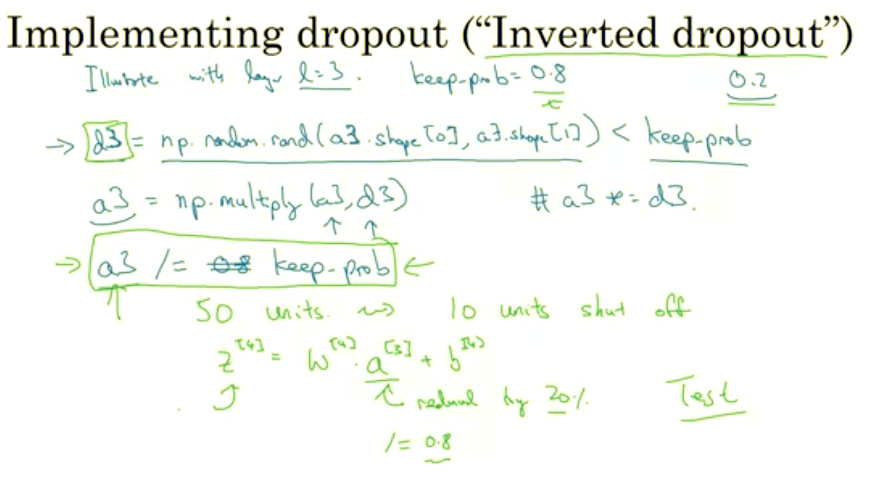and there will be the probability that a given hidden unit will be kept. So keep.prob = 0.8, then this means that there's a 0.2 chance of eliminating any hidden unit. So, what it does is it generates a random matrix. And this works as well if you have factorized. So d3 will be a matrix. Therefore, each example have a each hidden unit there's a 0.8 chance that the corresponding d3 will be one, and a 20% chance there will be zero. So, this random numbers being less than 0.8 it has a 0.8 chance of being one or be true, and 20% or 0.2 chance of being false, of being zero. And then what you are going to do is take your activations from the third layer, let me just call it a3 in this low example. So, a3 has the activations you computate. And you can set a3 to be equal to the old a3, times- There is element wise multiplication. Or you can also write this as a3* = d3. But what this does is for every element of d3 that's equal to zero. And there was a 20% ch ance of each of the elements being zero, just multiply operation ends up zeroing out, the corresponding element of d3. If you do this in python, technically d3 will be a boolean array where value is true and false, rather than one and zero. But the multiply operation works and will interpret the true and false values as one and zero. If you try this yourself in python, you'll see. Then finally, we're going to take a3 and scale it up by dividing by 0.8 or really dividing by our keep.prob parameter. So, let me explain what this final step is doing. Let's say for the sake of argument that you have 50 units or 50 neurons in the third hidden layer. So maybe a3 is 50 by one dimensional or if you- factorization maybe it's 50 by m dimensional. So, if you have a 80% chance of keeping them and 20% chance of eliminating them. This means that on average, you end up with 10 units shut off or 10 units zeroed out. And so now, if you look at the value of z^4, z^4 is going to be equal to $$w^4 * a^3 + b^4$$. And so, on expectation, this will be reduced by 20%. By which I mean that 20% of the elements of a3 will be zeroed out. So, in order to not reduce the expected value of z^4, what you do is you need to take this, and divide it by 0.8 because this will correct or just a bump that back up by roughly 20% that you need. So it's not changed the expected value of a3. And, so this line here is what's called the inverted dropout technique. And its effect is that, no matter what you set to keep.prob to, whether it's 0.8 or 0.9 or even one, if it's set to one then there's no dropout, because it's keeping everything or 0.5 or whatever, this inverted dropout technique by dividing by the keep.prob, it ensures that the expected value of a3 remains the same. And it turns out that at test time, when you trying to evaluate a neural network, which we'll talk about on the next slide, this inverted dropout technique, there is there is line to are due to the green box at dropping out. This makes test time easier because you have less of a scaling problem. By far the most common implementation of dropouts today as far as I know is inverted dropouts. I recommend you just implement this. But there were some early iterations of dropout that missed this divide by keep.prob line, and so at test time the average becomes more and more complicated. But again, people tend not to use those other versions. So, what you do is you use the d vector, and you'll notice that for different training examples, you zero out different hidden units. And in fact, if you make multiple passes through the same training set, then on different pauses through the training set, you should randomly zero out different hidden units. So, it's not that for one example, you should keep zeroing out the same hidden units is that, on iteration one of grade and descent, you might zero out some hidden units. And on the second iteration of great descent where you go through the training set the second time, maybe you'll zero out a different pattern of hidden units. And the vector d or d3, for the third layer, is used to decide what to zero out, both in for prob as well as in that prob. We are just showing for prob here. Now, having trained the algorithm at test time, here's what you would do. At test time, you're given some x or which you want to make a prediction. And using our standard notation, I'm going to use a^0, the activations of the zeroes layer to denote just test example x. So what we're going to do is not to use dropout at test time in particular which is in a sense. $$Z^1= w^1.a^0 + b^1. a^1 = g^1(z^1 Z). Z^2 = w^2.a^1 + b^2. a^2 =...$$ And so on. Until you get to the last layer and that you make a prediction y^. But notice that the test time you're not using dropout explicitly and you're not tossing coins at random, you're not flipping coins to decide which hidden units to eliminate. And that's because when you are making predictions at the test time, you don't really want your output to be random. If you are implementing dropout at test time, that just add noise to your predictions.

#### Introspect Results:

In theory, one thing you could do is run a prediction process many times with different hidden units randomly dropped out and have it across them. But that's computationally inefficient and will give you roughly the same result; very, very similar results to this different procedure as well. And just to mention, the inverted dropout thing, you remember the step on the previous line when we divided by the cheap.prob. The effect of that was to ensure that even when you don't see men dropout at test time to the scaling, the expected value of these activations don't change. So, you don't need to add in an extra funny scaling parameter at test time. That's different than when you have that training time. So that's dropouts. And when you implement this in week's premier exercise, you gain more firsthand experience with it as well. But why does it really work? What I want to do the next section is give you some better intuition about what dropout really is doing. Let's go on to the next section.

## Understanding Dropout

Drop out does this seemingly crazy thing of randomly knocking out units on your network. Why does it work so well with a regularizer? Let's gain some better intuition. In the previous section, I gave this intuition that drop-out randomly knocks out units in your network. So it's as if on every iteration you're working with a smaller neural network, and so using a smaller neural network seems like it should have a regularizing effect.

#### Why Does Dropout Work ?

Here's a second intuition which is, let's look at it from the perspective of a single unit. Let's say this one. Now, for this unit to do his job as for inputs and it needs to generate some meaningful output. Now with drop out, the inputs can get randomly eliminated. Sometimes those two units will get eliminated, sometimes a different unit will get eliminated.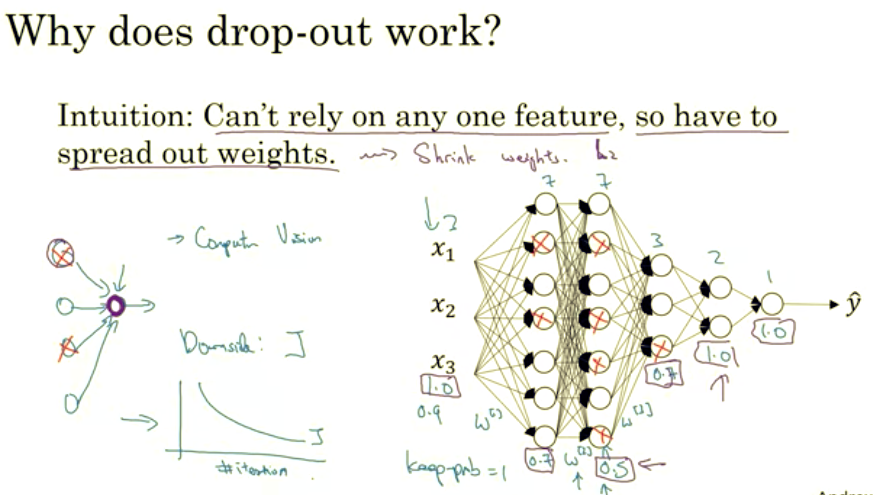So, what this means is that this unit, which I'm circling in purple, it can't rely on any one feature because any one feature could go away at random or any one of its own inputs could go away at random. Some particular would be reluctant to put all of its bets on, say, just this input, right? The weights, we're reluctant to put too much weight on any one input because it can go away. So this unit will be more motivated to spread out this way and give you a little bit of weight to each of the four inputs to this unit. And by spreading all the weights, this will tend to have an effect of shrinking the squared norm of the weights. And so, similar to what we saw with L2 regularization, the effect of implementing drop out is that it shrinks the weights and does some of those outer regularization that helps prevent over-fitting. But it turns out that drop out can formally be shown to be an adaptive form without a regularization. But L2 penalty on different weights are different, depending on the size of the activations being multiplied that way. But to summarize, it is possible to show that drop out has a similar effect to L2 regularization. Only to L2 regularization applied to different ways can be a little bit different and even more adaptive to the scale of different inputs. One more detail for when you're implementing drop out. Here's a network where you have three input features. This is seven hidden units here, seven, three, two, one. So, one of the parameters we had to choose was the cheap prop which has a chance of keeping a unit in each layer. So, it is also feasible to vary key prop by layer. So for the first layer, your matrix W1 will be three by seven. Your second weight matrix will be seven by seven. W3 will be seven by three and so on. And so W2 is actually the biggest weight matrix, because they're actually the largest set of parameters would be in W2 which is seven by seven. So to prevent, to reduce over-fitting of that matrix, maybe for this layer, I guess this is layer two, you might have a key prop that's relatively low, say zero point five, whereas for different layers where you might worry less about over-fitting, you could have a higher key prop, maybe just zero point seven. And if a layers we don't worry about over-fitting at all, you can have a key prop of one point zero.

#### For clarity

These numbers I'm drawing on the purple boxes, These could be different key props for different layers. Notice that the key prop of one point zero means that you're keeping every unit and so, you're really not using drop out for that layer. But for layers where you're more worried about over-fitting, really the layers with a lot of parameters, you can set the key prop to be smaller to apply a more powerful form of drop out. It's kind of like cranking up the regularization parameter lambda of L2 regularization where you try to regularize some layers more than others. And technically, you can also apply drop out to the input layer, where you can have some chance of just maxing out one or more of the input features. Although in practice, usually don't do that that often. And so, a key prop of one point zero was quite common for the input there. You can also use a very high value, maybe zero point nine, but it's much less likely that you want to eliminate half of the input features. So usually key prop, if you apply the law, will be a number close to one if you even apply drop out at all to the input there. So just to summarize, if you're more worried about some layers overfitting than others, you can set a lower key prop for some layers than others.

### Downside of Dropout

The downside is, this gives you even more hyper parameters to search for using cross-validation. One other alternative might be to have some layers where you apply drop out and some layers where you don't apply drop out and then just have one hyper parameter, which is a key prop for the layers for which you do apply drop outs. And before we wrap up, just a couple implementational tips. Many of the first successful implementations of drop outs were to computer vision. So in computer vision, the input size is so big, inputting all these pixels that you almost never have enough data. And so drop out is very frequently used by computer vision. And there's some computer vision researchers that pretty much always use it, almost as a default. But really the thing to remember is that drop out is a regularization technique, it helps prevent over-fitting. And so, unless my algorithm is over-fitting, I wouldn't actually bother to use drop out. So it's used somewhat less often than other application areas. There's just with computer vision, you usually just don't have enough data, so you're almost always overfitting, which is why there tends to be some computer vision researchers who swear by drop out. But their intuition doesn't always generalize I think to other disciplines. One big downside of drop out is that the cost function J is no longer well-defined. On every iteration, you are randomly killing off a bunch of nodes. And so, if you are double checking the performance of grade and dissent, it's actually harder to double check that you have a well defined cost function J that is going downhill on every iteration. Because the cost function J that you're optimizing is actually less. Less well defined, or is certainly hard to calculate.

So you lose this debugging tool to will a plot, a graph like this. So what I usually do is turn off drop out, you will set key prop equals one, and I run my code and make sure that it is monotonically decreasing J, and then turn on drop out and hope that I didn’t introduce bugs into my code during drop out. Because you need other ways, I guess, but not plotting these figures to make sure that your code is working to greatness and it’s working even with drop outs. So with that, there’s still a few more regularization techniques that are worth your knowing. Let’s talk about a few more such techniques in the next section.

## Other regularization methods

In addition to L2 regularization and drop out regularization there are few other techniques to reducing over fitting in your neural network.

### Data Augmentation

Let's take a look. Let's say you fitting a CAD crossfire. If you are over fitting getting more training data can help, but getting more training data can be expensive and sometimes you just can't get more data. But what you can do is augment your training set by taking image like this.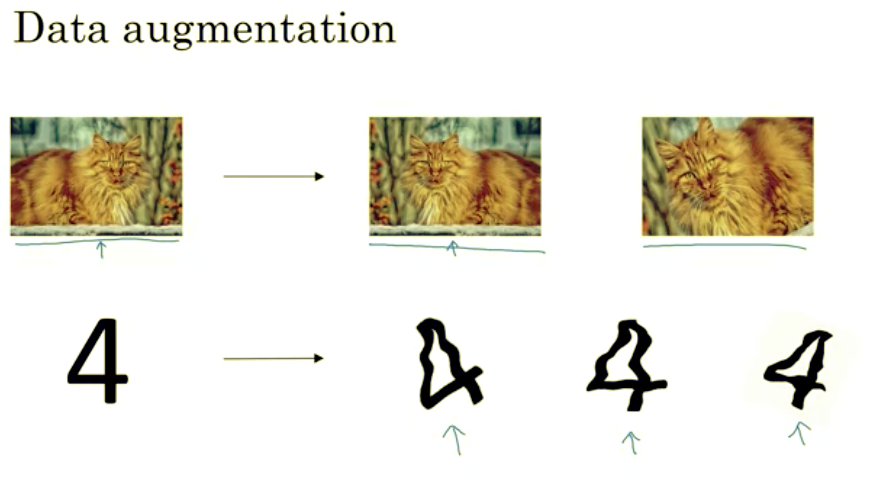### Early Stopping

There's one other technique that is often used called early stopping. So what you're going to do is as you run gradient descent you're going to plot your, either the training error, you'll use 01 classification error on the training set. Or just plot the cost function J optimizing, and that should decrease monotonically, like so, all right? Because as you trade, hopefully, you're trading around your cost function J should decrease.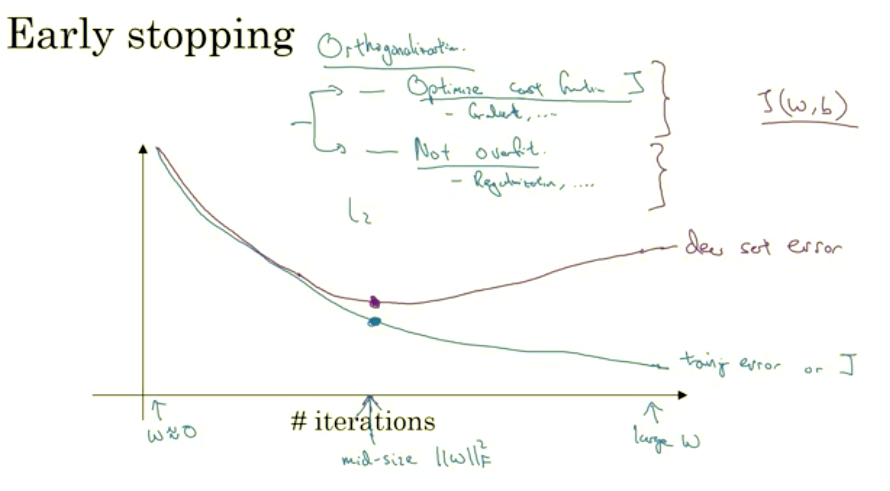So with early stopping, what you do is you plot this, and you also plot your dev set error. And again, this could be a classification error in a development sense, or something like the cost function, like the logistic loss or the log loss of the dev set. Now what you find is that your dev set error will usually go down for a while, and then it will increase from there. So what early stopping does is, you will say well, it looks like your neural network was doing best around that iteration, so we just want to stop trading on your neural network halfway and take whatever value achieved this dev set error. So why does this work? Well when you've haven't run many iterations for your neural network yet your parameters w will be close to zero. Because with random initialization you probably initialize w to small random values so before you train for a long time, w is still quite small. And as you iterate, as you train, w will get bigger and bigger and bigger until here maybe you have a much larger value of the parameters w for your neural network. So what early stopping does is by stopping halfway you have only a mid-size rate w.

And so similar to L2 regularization by picking a neural network with smaller norm for your parameters w, hopefully your neural network is over fitting less. And the term early stopping refers to the fact that you’re just stopping the training of your neural network earlier.